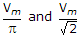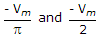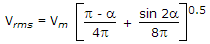# Electronics and Communication Engineering - Power Electronics

Exercise : Power Electronics - Section 1
36.
A single phase half wave controlled rectifier circuit has a free wheeling diode. The load is a combination of R and L. The firing angle is a. The period of conduction of SCR and free wheeling diode respectively are
a < ωt < p and p < ωt < (2p + a)
a < ωt < p and p < ωt < 2p
a < ωt < (p + a) and (p + a) < ωt < 2p
a < ωt < p and p < ωt < (2p - a)
Explanation:

SCR conducts from a to p and free wheeling diode from p to (2p + a).

37.

Assertion (A): A transistor requires a continuous base signal for conduction but a thyristor requires a gate pulse.

Reason (R): Transistor find widespread application in power electronic circuits.

Both A and R are correct and R is correct explanation of A
Both A and R correct but R is not correct explanation of A
A is correct but R is wrong
A is wrong but R is correct
Explanation:

Transistors are used but not very widely.

38.
In a thyristor the gate current is increased, then
anode current will increase
anode current will decrease
anode current will remain constant
anode current may increase or decrease
Explanation:

Gate current does not affect the magnitude of anode current.

39.
Thyristors are not suitable for logic circuits.
True
False
Explanation:

Thyristors can be used for logic circuits.

40.
A single phase half wave rectifier is feeding a resistive load. Input voltage v = Vm sin ωt. The output dc voltage is Vdc and output rms voltage is Vrms . If firing angle is 180°, Vdc and Vrms respectively are
0 and 00 and - VmPut a = 180° in Eqs